Courses

# Test: Previous Year Questions: Ionic Equilibrium

## 15 Questions MCQ Test Chemistry for JEE | Test: Previous Year Questions: Ionic Equilibrium

Description
This mock test of Test: Previous Year Questions: Ionic Equilibrium for JEE helps you for every JEE entrance exam. This contains 15 Multiple Choice Questions for JEE Test: Previous Year Questions: Ionic Equilibrium (mcq) to study with solutions a complete question bank. The solved questions answers in this Test: Previous Year Questions: Ionic Equilibrium quiz give you a good mix of easy questions and tough questions. JEE students definitely take this Test: Previous Year Questions: Ionic Equilibrium exercise for a better result in the exam. You can find other Test: Previous Year Questions: Ionic Equilibrium extra questions, long questions & short questions for JEE on EduRev as well by searching above.
QUESTION: 1

### The solubility of Mg(OH)2 is x mole/ltr. then its solubility product is -  [AIEEE-2002]

Solution: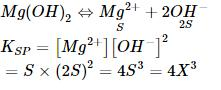QUESTION: 2

### The solubility in water of a sparingly soluble salt AB2 is 1.0 × 10-5 mol L-1 . Its solubility product will be - [AIEEE-2003]

Solution:

AB2                A       +     2B
S                     S                2S
Ksp = [A][B]2
= s (2s)2
1.0×10-5×(2×1.0×10-5)2
= 4*10-15

QUESTION: 3

### The molar solubility (in mol L-1) of a sparingly soluble salt MX4 is `s'. The corresponding solubility product is KSP. `s' is given in terms of Ksp by the relation - [AIEEE-2004]

Solution: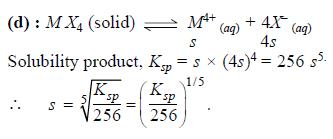QUESTION: 4

The solubility product of a salt having general formula MX2, in water is 4x10-12. The concentration of M2+ ions in the aqueous solution of the salt is - [AIEEE-2005]

Solution: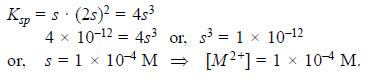QUESTION: 5

The first and second dissociation constants of an acid H2A are 1.0 x 10-5 & 5.0 x 10-10 respectively. The overall dissociation constant of the acid will be -

[AIEEE-2007]

Solution: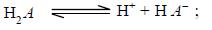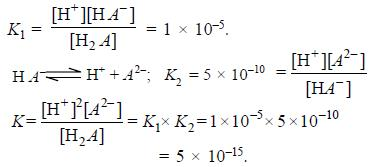QUESTION: 6

The pKa of a weak acid (HA) is 4.5. The pOH of an aqueous buffered solution of HA in which 50% of the acid is ionized is-          [AIEEE-2007]

Solution:

For buffer solution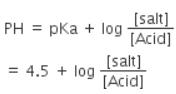as HA is 50% ionized so [Salt] = [Acid]
pH = 4.5 pH + pOH = 14
⇒ pOH = 14 – 4.5 = 9.5

QUESTION: 7

In a saturated solution of the sparingly soluble strong electrolyte AgIO3 (molecular mass = 283) the equilibrium which sets in is -

AgIO3(s)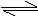Ag+(aq) + IO3¯(aq)

If the solubility product Ksp of AgIO3 at a given temperature is 1.0 x 10-8, what is the mass of AgIO3 contained in 100 ml of its saturated solution ?

[AIEEE-2007]

Solution: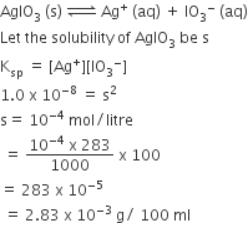QUESTION: 8

The pKa of a weak acid, HA, is 4.80. The pKb of a weak base, BOH, is 4.78. The pH of an aqueous solution of the corresponding salt, BA, will be -

[AIEEE-2008]

Solution: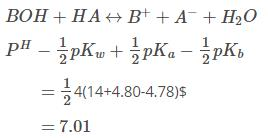QUESTION: 9

Solid Ba(NO3)2 is gradually dissolved in a 1.0 x 10-4 M Na2CO3 solution. At what concentration of Ba2+ will a precipitate begin to form ? (Ksp for Ba CO3 = 5.1 x 10-9)

[AIEEE-2009]

Solution: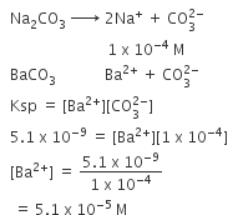QUESTION: 10

In aqueous solution the ionization constants for carbonic acid are K1 = 4.2 x 10-7 and K2 = 4.8 x 10-11 Selection the correct statement for a saturated 0.034 M solution of the carbonic acid.

[AIEEE-2010]

Solution:

Carbonic acid is a weak acid it dissociates as follows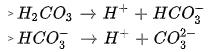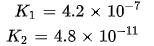Since both acids are weak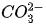cannot be 0.034 M as there is no complete dissociation.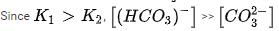Total hydrogen ion concentration is approximately equal to concentration of hydrogen ion in first reaction as K1 is larger. This is approximately equal to concentration ofThe concentration ofdepends onand that ofon. But. So The concentration ofis not double that ofQUESTION: 11

Solubility product of silver bromide is 5.0 x 10-13. The quantity of potassium bromide (molar mass taken as 120 g mol-1) to be added to 1 litre of 0.05 M solution of silver nitrate to start the precipitation of AgBr is

[AIEEE-2010]

Solution:

For precipitation

[Ag+][Br-] > Ksp[AgBr]

[Br-]min = (5 x 10-13)/0.05 = 10-11 M

Mass of potassium bromide needed = 10-11 x 120

= 1.2 x 10-9 g

QUESTION: 12

Three reactions involving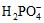are given below:

(i)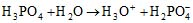(ii)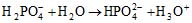(iii)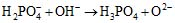In which of the above doesact as an acid?

[AIEEE-2010]

Solution:

Acids are those substances which gives H+. Here in reaction ii only, H2PO4- acts as an acid.

QUESTION: 13

At 25°C, the solubility product of Mg(OH)2 is 1.0 ×10-11. At which pH, will Mg2+ ions start precipitating in the form of Mg(OH)2 from a solution of 0.001 M Mg2+ions ?

[AIEEE-2010]

Solution: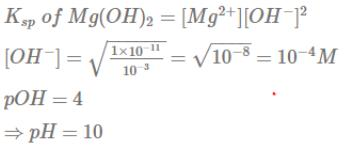QUESTION: 14

The pH of a 0.1 molar solution of the acid HQ is 3. The value of the ionization constant, Ka of this acid is -

[AIEEE-2012]

Solution: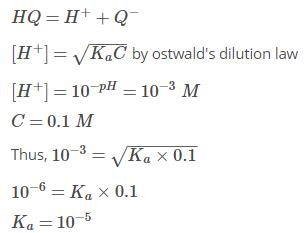QUESTION: 15

How many litres of water must be added to litre of an aqueous solution of HCl with a pH of 1 to create an aqueous solution with pH of 2?

[AIEEE-2013]

Solution:

Volume of the original solution = 1 L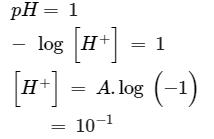pH of the new solution = 2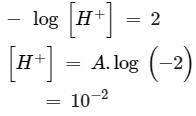Volume of the new solution = ?
As per volumetric principle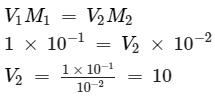That means the volume of water added to the original solution of 1 L = 10 −1 = 9 L